Trigonometric Values: Unit Circle Form

what you'll learn...

Names of Trigonometric Functions

»  Angle $\theta$$\theta$ specifies a class of similar right-triangles.

→  Any one representative triangle captures the properties of all in the class.

»  The representative triangle is chosen in the unit circle.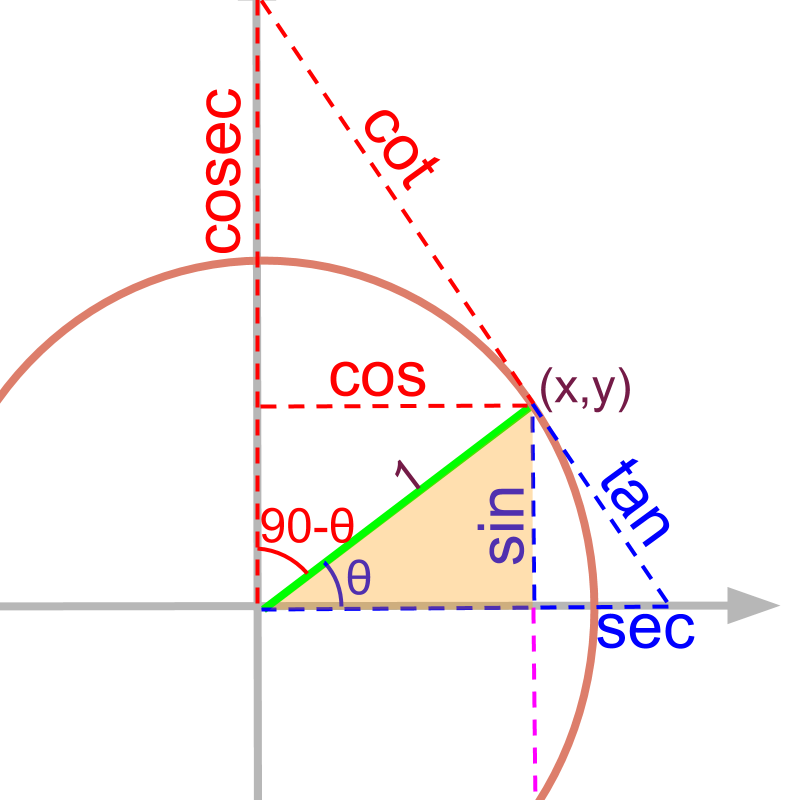»  sine: root word meaning chord.
»  tangent: meaning touching the curve
»  secant: meaning cutting through

»  complementary: meaning completes to right angle.
»  co-sine
»  co-tangent
»  co-secant

Trigonometric Values Redefined

»  Point on unit circle at the given angle $\theta$$\theta$
Representative of the class of similar right-triangles

»  Projection on y-axis = $y$$y$
→  opposite side in triangle is generalized to the y-coordinate

»  Projection on x-axis = $x$$x$
→  adjacent side in triangle is generalized to the x-coordinate

»  $\mathrm{sin}\theta =y$$\sin \theta = y$
y-coordinate of the point on unit circle

»  $\mathrm{cos}\theta =x$$\cos \theta = x$
x-coordinate of the point on unit circle

»  and accordingly $\mathrm{tan}$$\tan$, $\mathrm{sec}$$\sec$, $\mathrm{csc}$$\csc$, $\mathrm{cot}$$\cot$.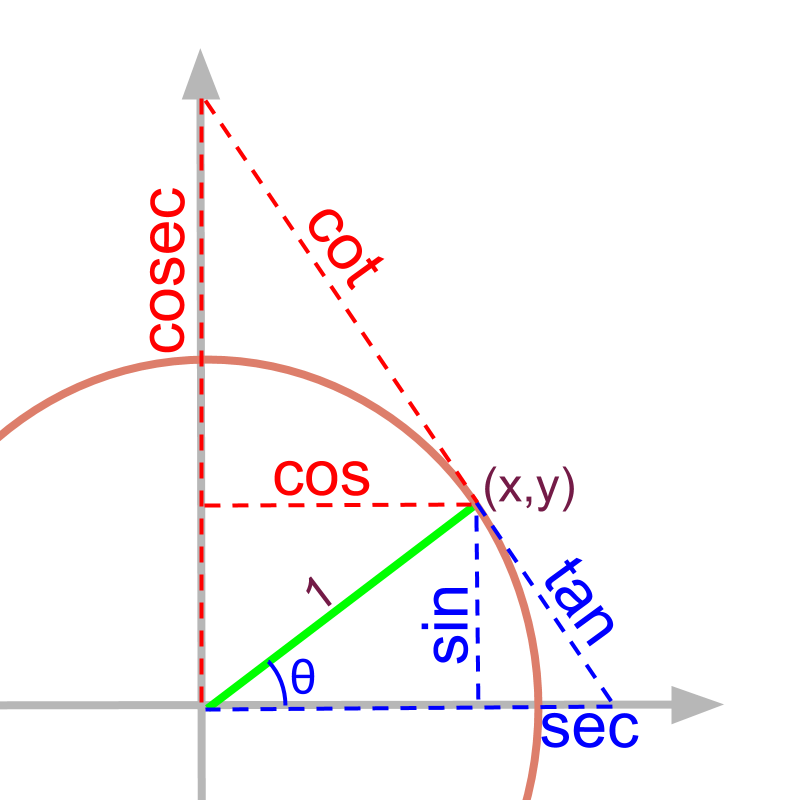recap

When starting trigonometry, the definitions of trigonometric ratios were explained for right angled triangles.

•  sine,

•  cosine,

•  tan (tangent),

•  secant,

•  co-secant, and

•  cot (co-tangent)

Let us look at a refined definition of the same.

unit circle

A circle with radius $1$$1$ unit is called an unit circle.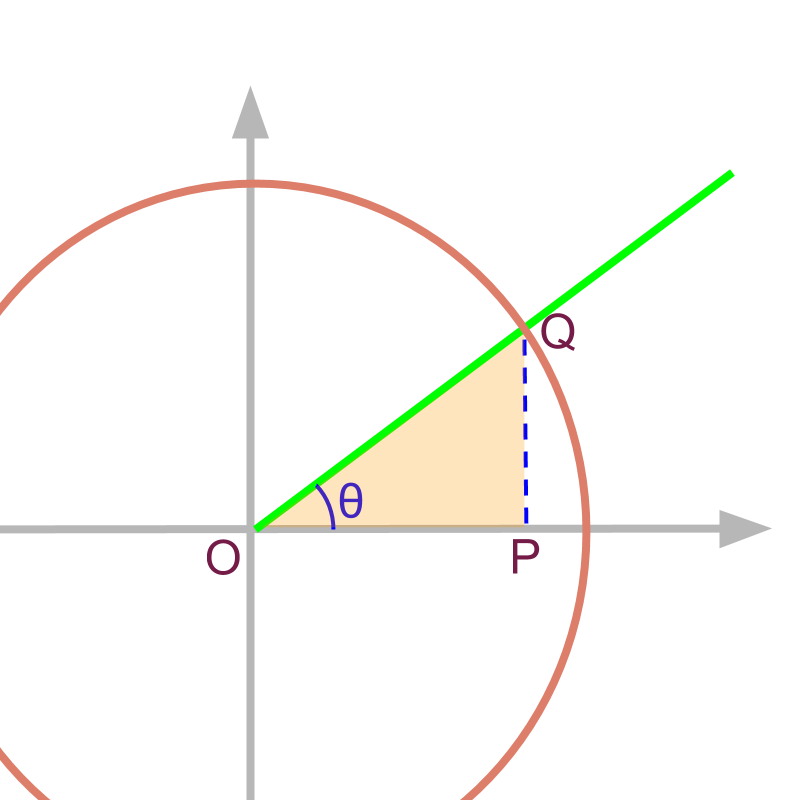It was explained that trigonometric ratios are defined for set of similar right angled triangles. Consider the right angled triangle made within a unit circle as given in the figure. Any right angled triangle with one angle $\theta$$\theta$ is represented by the $△OPQ$$\triangle O P Q$.

The hypotenuse in the given triangle is $\overline{OQ}$$\overline{O Q}$.

The $\overline{OP}$$\overline{O P}$ is the adjacent side and $\overline{PQ}$$\overline{P Q}$ is the opposite side to $\angle \theta$$\angle \theta$

chord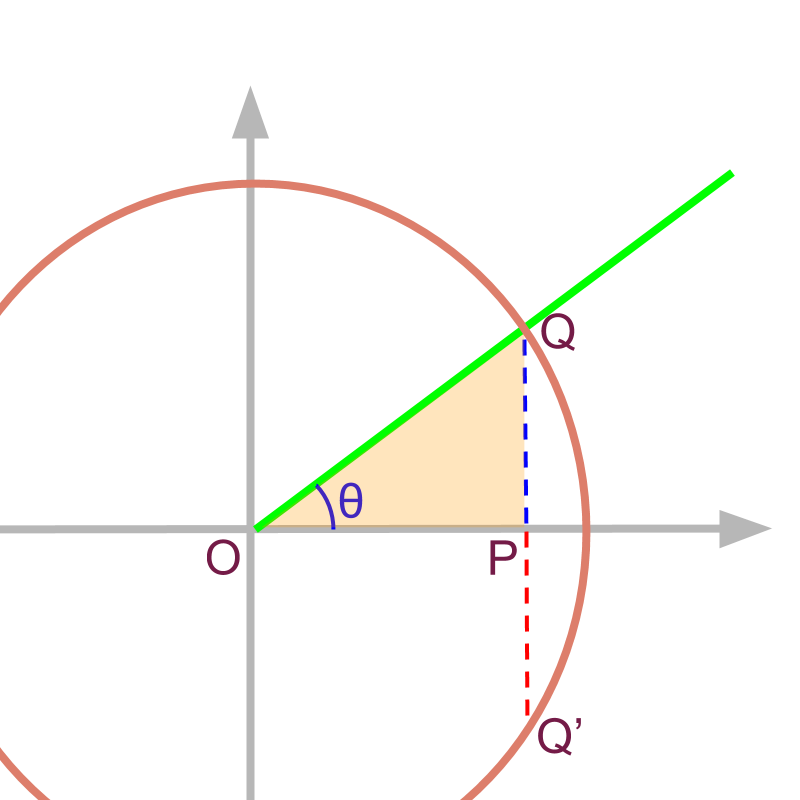The chord to the circle is $\overline{\left(QQ\prime \right)}$.

Note that in the given unit circle $\overline{OQ}=1$$\overline{O Q} = 1$.

$\mathrm{sin}\theta$$\sin \theta$ in the given figure is $\overline{PQ}÷\overline{OQ}$$\overline{P Q} \div \overline{O Q}$ $=\overline{PQ}$$= \overline{P Q}$

The root word of $\mathrm{sin}$$\sin$ refers to chord of a circle.

For a given angle $\theta$$\theta$, the line $\overline{PQ}$$\overline{P Q}$ is half of the chord as shown in figure. So the ratio is named as $\mathrm{sin}$$\sin$ referring the relation to length of the chord at the given angle.

redefine sine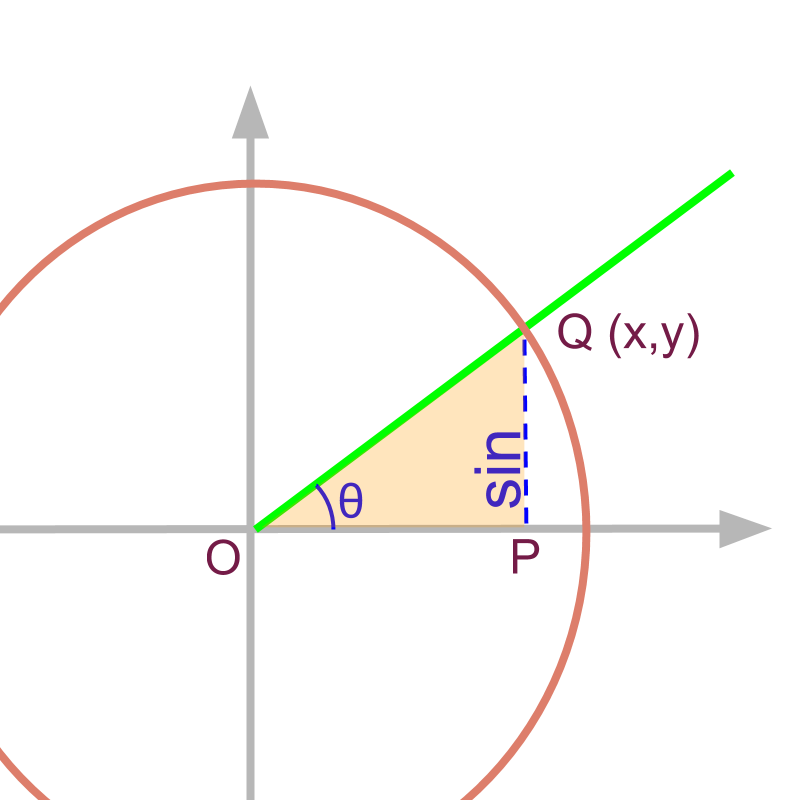Given that the point $Q$$Q$ is $\left(x,y\right)$$\left(x , y\right)$,

$\mathrm{sin}\theta =y$$\sin \theta = y$

So far, $\mathrm{sin},\mathrm{cos},...$$\sin , \cos , \ldots$ were referred as trigonometric ratios. Considering the unit circle and the point $\left(x,y\right)$$\left(x , y\right)$ at angle $\theta$$\theta$ on the unit circle, $\mathrm{sin},\mathrm{cos}...$$\sin , \cos \ldots$ are referred as trigonometric values.

$\mathrm{sin}\theta$$\sin \theta$ is the projection on y-axis for the line of unit length at angle $\theta$$\theta$.

complementary

The complementary angle of an angle $\theta$$\theta$ is $90-\theta$$90 - \theta$.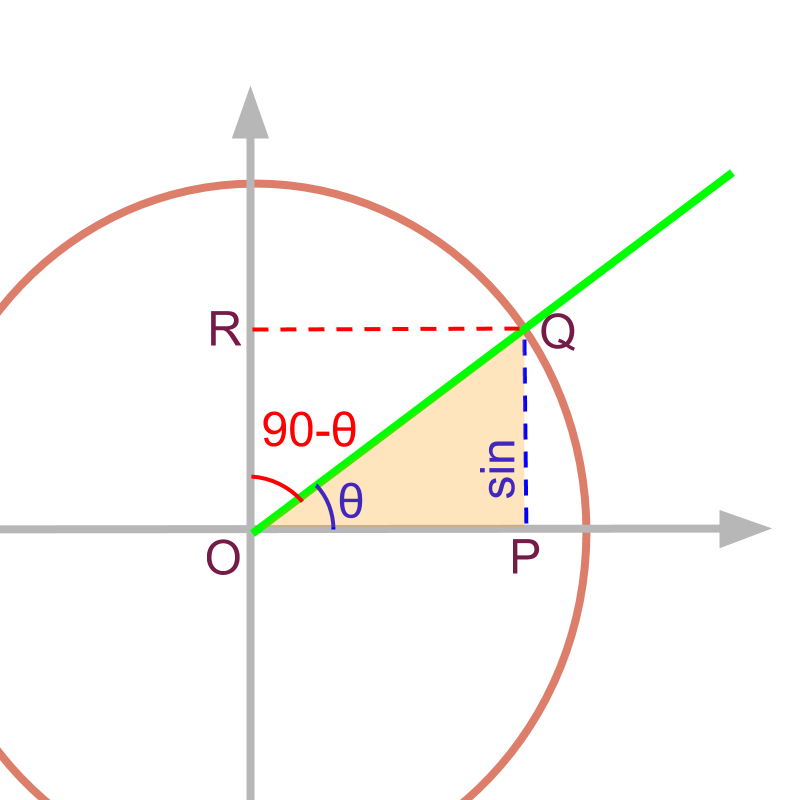The complementary angle for $\angle POQ=\theta$$\angle P O Q = \theta$ is $\angle QOR$$\angle Q O R$.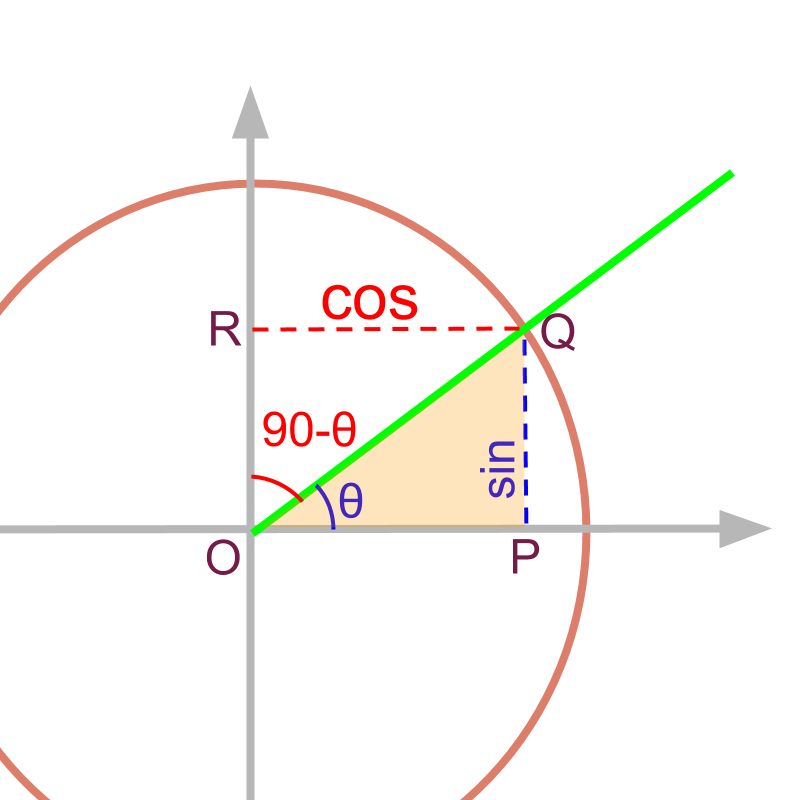For a given angle $\theta$$\theta$, the $\mathrm{sin}$$\sin$ of complementary angle is $\mathrm{cos}$$\cos$ or cosine.

co-sine is the short form of 'complementary sine'.

redefine cosine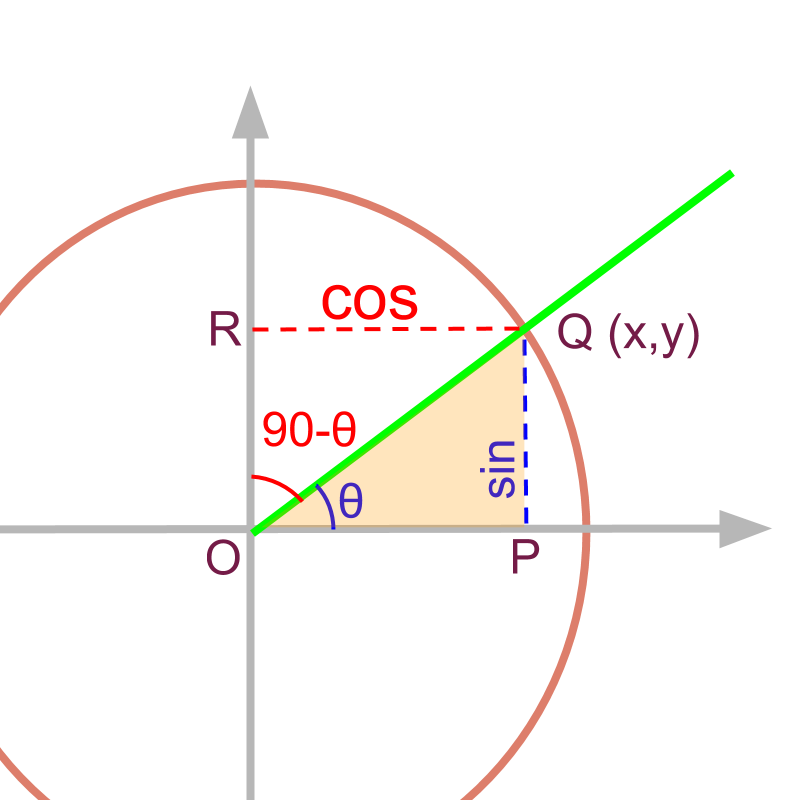Given that the point $Q$$Q$ is $\left(x,y\right)$$\left(x , y\right)$,

$\mathrm{cos}\theta =x$$\cos \theta = x$

$\mathrm{cos}\theta$$\cos \theta$ is the projection on x-axis for the line of unit length at angle $\theta$$\theta$.

line touching the circle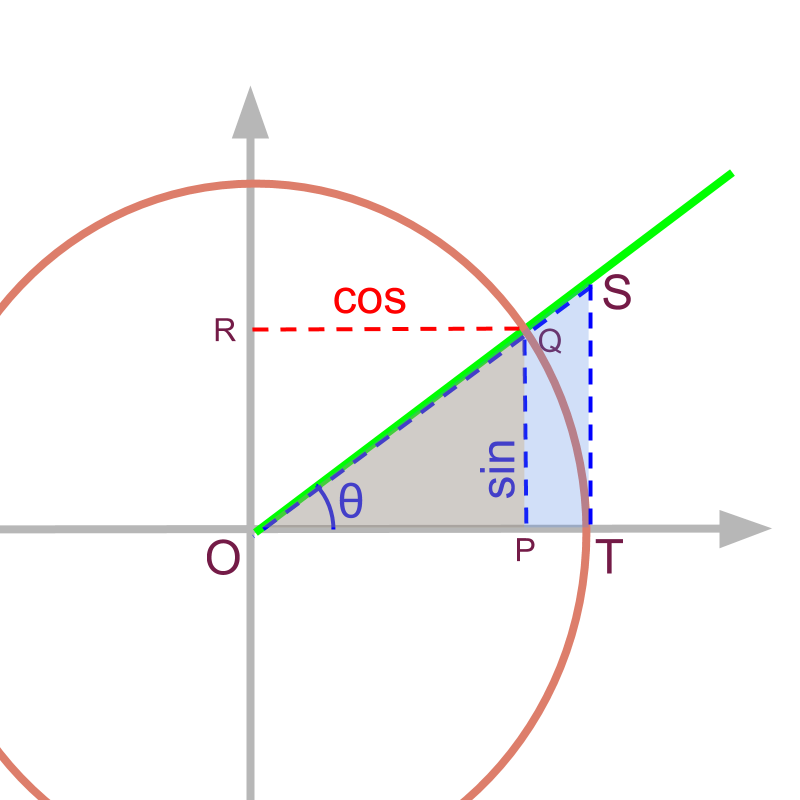Consider two triangles $OPQ$$O P Q$ and $OTS$$O T S$. It is noted that $\overline{TS}$$\overline{T S}$ is a tangent to the circle.

The triangles $△OPQ$$\triangle O P Q$ and $△OTS$$\triangle O T S$ are similar right-angled-triangles. And $\overline{OT}=\overline{OQ}=1$$\overline{O T} = \overline{O Q} = 1$ as it is unit circle.

ratios of sides of similar triangles are equal
$\overline{ST}÷\overline{OT}=\overline{PQ}÷\overline{OP}$$\overline{S T} \div \overline{O T} = \overline{P Q} \div \overline{O P}$

substituting $\overline{OT}=1$$\overline{O T} = 1$, $\overline{PQ}=\mathrm{sin}\theta$$\overline{P Q} = \sin \theta$ and $\overline{OP}=\mathrm{cos}\theta$$\overline{O P} = \cos \theta$
$\overline{ST}=\frac{\mathrm{sin}\theta }{\mathrm{cos}\theta }$$\overline{S T} = \frac{\sin \theta}{\cos \theta}$
$\overline{ST}=\mathrm{tan}\theta$$\overline{S T} = \tan \theta$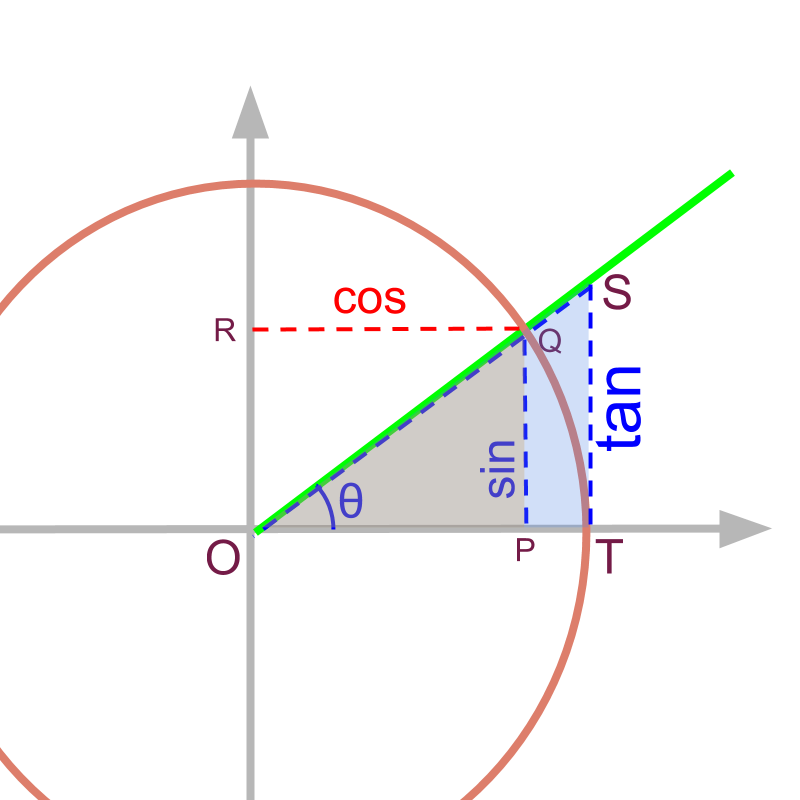For a given angle $\theta$$\theta$, the $\mathrm{tan}\theta$$\tan \theta$ is the length of the line segment on tangent as shown in figure.

$\mathrm{tan}$$\tan$ is the short form of 'tangent'.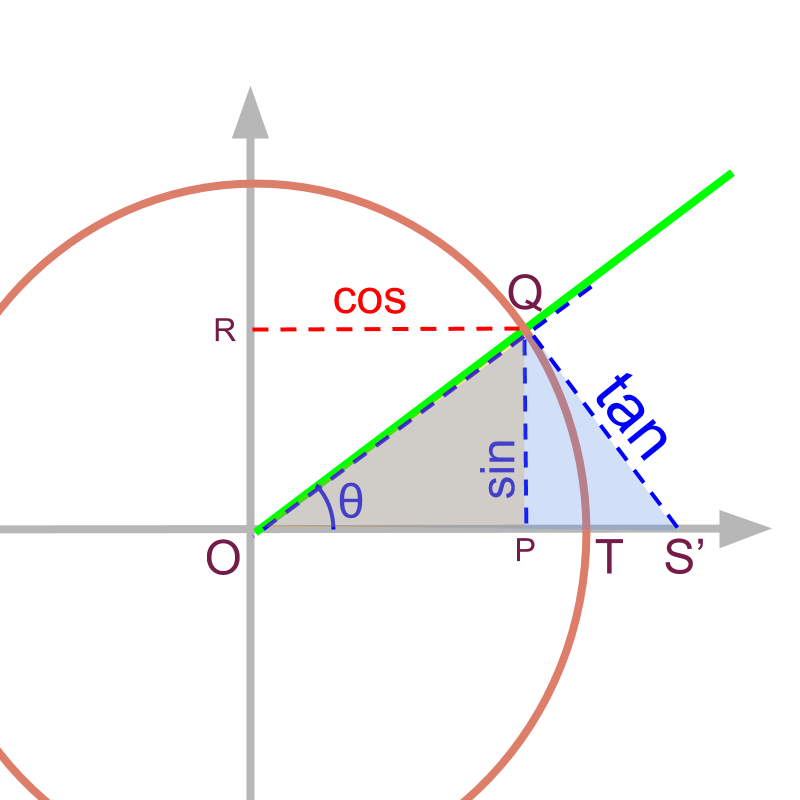The $\mathrm{tan}$$\tan$ of an angle is equivalently given as $\overline{QS\prime }$ as shown in figure.

Note the following:
$△OPQ$$\triangle O P Q$ and $△OQS\prime$ are similar triangles and so
$\frac{\overline{QS\prime }}{\overline{OQ}}=\frac{\overline{PQ}}{\overline{OP}}$
so, $\mathrm{tan}\theta =\frac{\overline{PQ}}{\overline{OP}}$$\tan \theta = \frac{\overline{P Q}}{\overline{O P}}$

line cutting the circle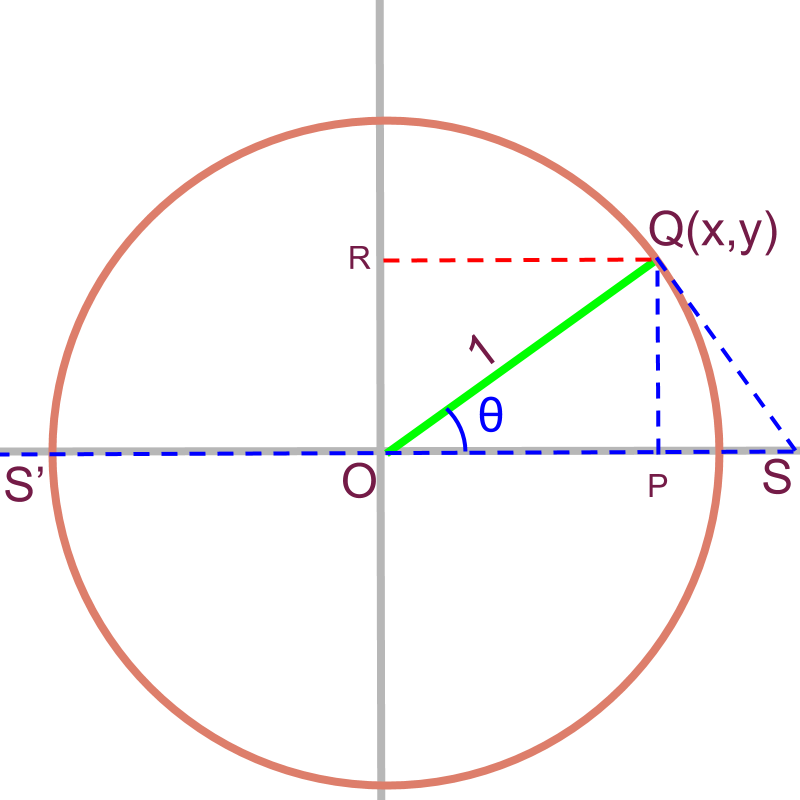Consider the line $\overline{S\prime S}$. It is a secant to the circle.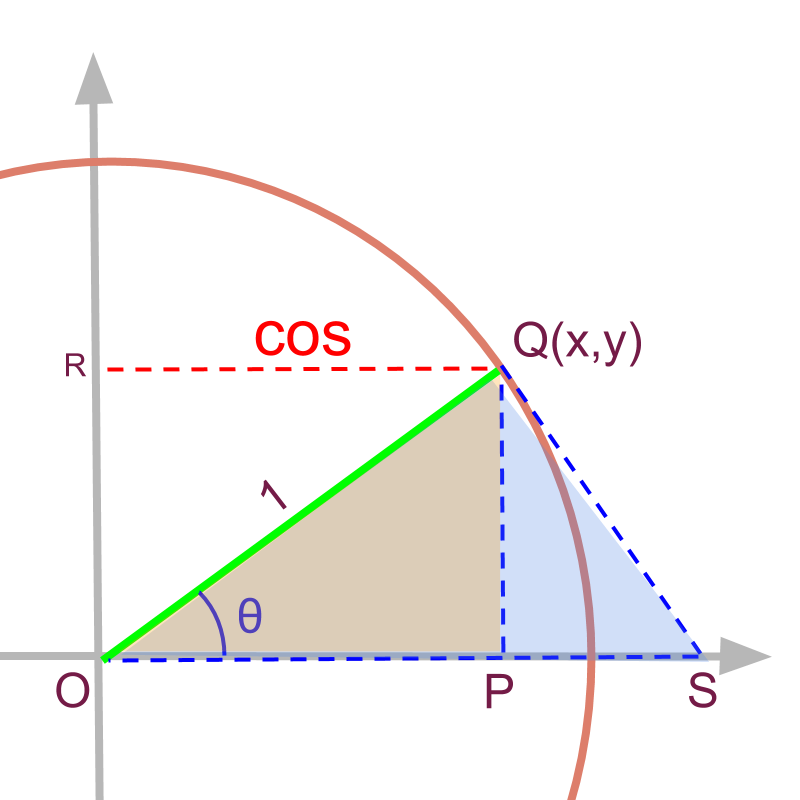Consider the circle with part of the secant $OS$$O S$

$△OPQ$$\triangle O P Q$ and $△OQS$$\triangle O Q S$ are similar right angled triangles.
So $\text{hypotenuse}÷\text{adjacent}$$\textrm{h y p o t e \nu s e} \div \textrm{a \mathrm{dj} a c e n t}$ for the triangles are equal.

$\overline{OS}÷\overline{OQ}=\overline{OQ}÷\overline{OP}$$\overline{O S} \div \overline{O Q} = \overline{O Q} \div \overline{O P}$

Substitute $\overline{OQ}=1$$\overline{O Q} = 1$, and $\overline{OP}=\mathrm{cos}\theta$$\overline{O P} = \cos \theta$

the line segment
$\overline{OS}$$\overline{O S}$
$=\frac{1}{\mathrm{cos}\theta }$$= 1 \frac{\setminus}{\cos \theta}$
$=\mathrm{sec}\theta$$= \sec \theta$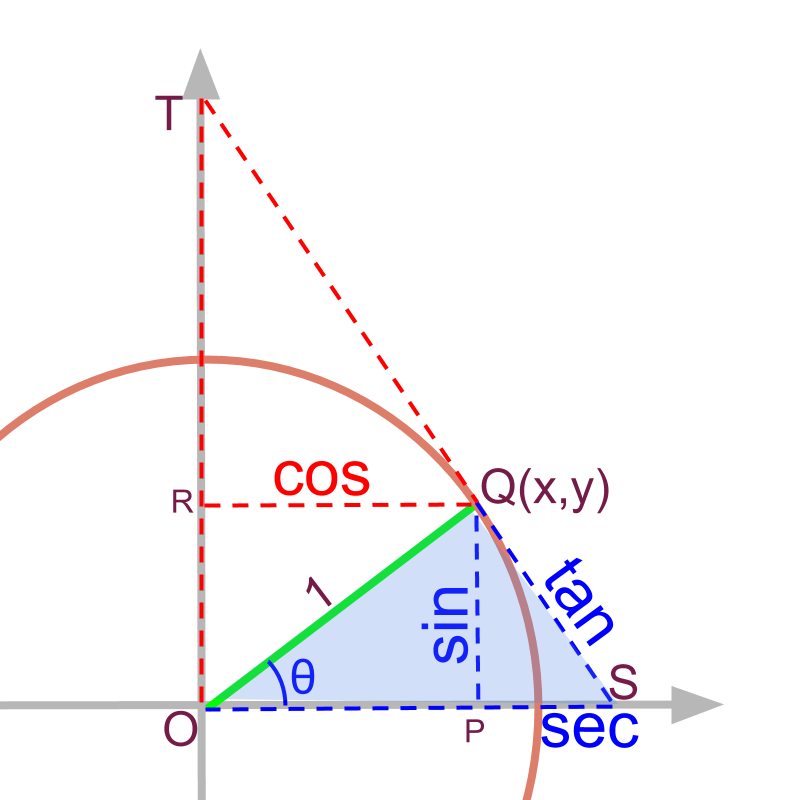The trigonometric value corresponding to $\overline{OS}$$\overline{O S}$ is $\mathrm{sec}\theta$$\sec \theta$.

$\mathrm{sec}\theta =\frac{1}{\mathrm{cos}\theta }=\frac{1}{x}$$\sec \theta = \frac{1}{\cos \theta} = \frac{1}{x}$

cosecant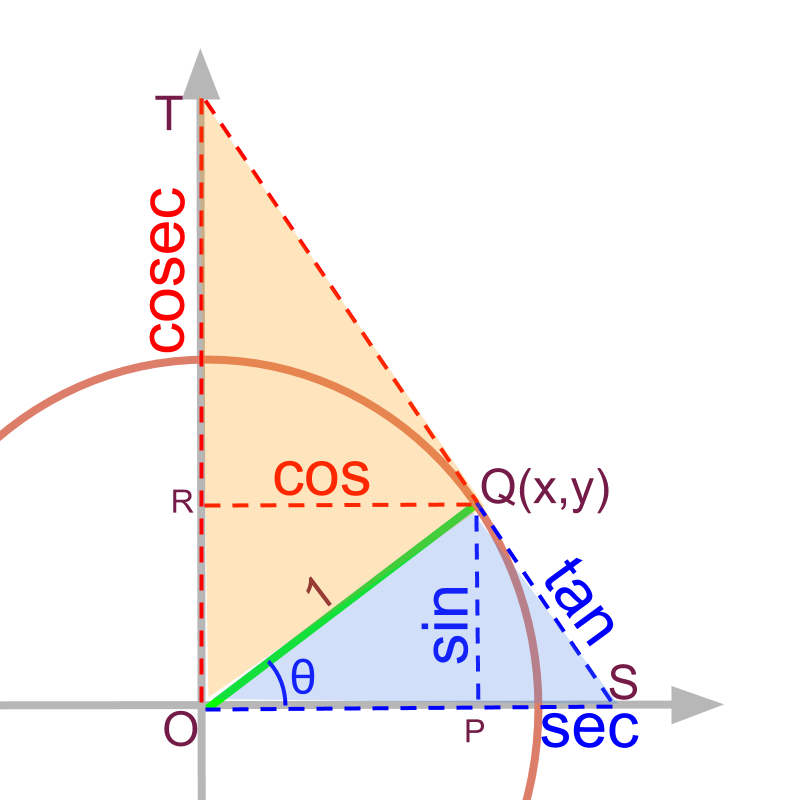The secant for the complementary angle of theta is $\overline{OT}$$\overline{O T}$ as given in the figure. It is noted that $△TQO$$\triangle T Q O$ and $△OPQ$$\triangle O P Q$ are similar right-angled-triangles.

Ratio of corresponding sides are equal $\frac{\overline{OT}}{\overline{OQ}}=\frac{\overline{OQ}}{\overline{PQ}}$$\frac{\overline{O T}}{\overline{O Q}} = \frac{\overline{O Q}}{\overline{P Q}}$

substitute $\overline{OQ}=1$$\overline{O Q} = 1$ and $\overline{PQ}=\mathrm{sin}\theta$$\overline{P Q} = \sin \theta$

The line segment $\overline{OT}$$\overline{O T}$

$=\frac{1}{\mathrm{sin}\theta }$$= \frac{1}{\sin \theta}$

$=\text{cosec}\theta \phantom{\rule{1ex}{0ex}}\text{or}\phantom{\rule{1ex}{0ex}}\mathrm{csc}\theta$$= \textrm{\cos e c} \theta \textrm{\mathmr{and}} \csc \theta$

The trigonometric value corresponding to $\overline{OT}$$\overline{O T}$ is $\text{cosec}\theta$$\textrm{\cos e c} \theta$.

$\text{cosec}\theta =\frac{1}{\mathrm{sin}\theta }=\frac{1}{y}$$\textrm{\cos e c} \theta = \frac{1}{\sin \theta} = \frac{1}{y}$

cotangent

The tangent for the complementary angle of theta is $\overline{QT}$$\overline{Q T}$ as given in the figure.

It is noted that $△TQO$$\triangle T Q O$ and $△OPQ$$\triangle O P Q$ are similar right-angled-triangles.

ratio of corresponding sides are equal $\frac{\overline{QT}}{\overline{OQ}}=\frac{\overline{OP}}{\overline{PQ}}$$\frac{\overline{Q T}}{\overline{O Q}} = \frac{\overline{O P}}{\overline{P Q}}$

substitute $\overline{OQ}=1$$\overline{O Q} = 1$, $\overline{PQ}=\mathrm{sin}\theta$$\overline{P Q} = \sin \theta$ and $\overline{OP}=\mathrm{cos}\theta$$\overline{O P} = \cos \theta$.

The $\overline{QT}$$\overline{Q T}$

$=\frac{\mathrm{cos}\theta }{\mathrm{sin}\theta }$$= \frac{\cos \theta}{\sin \theta}$

$=\mathrm{cot}\theta$$= \cot \theta$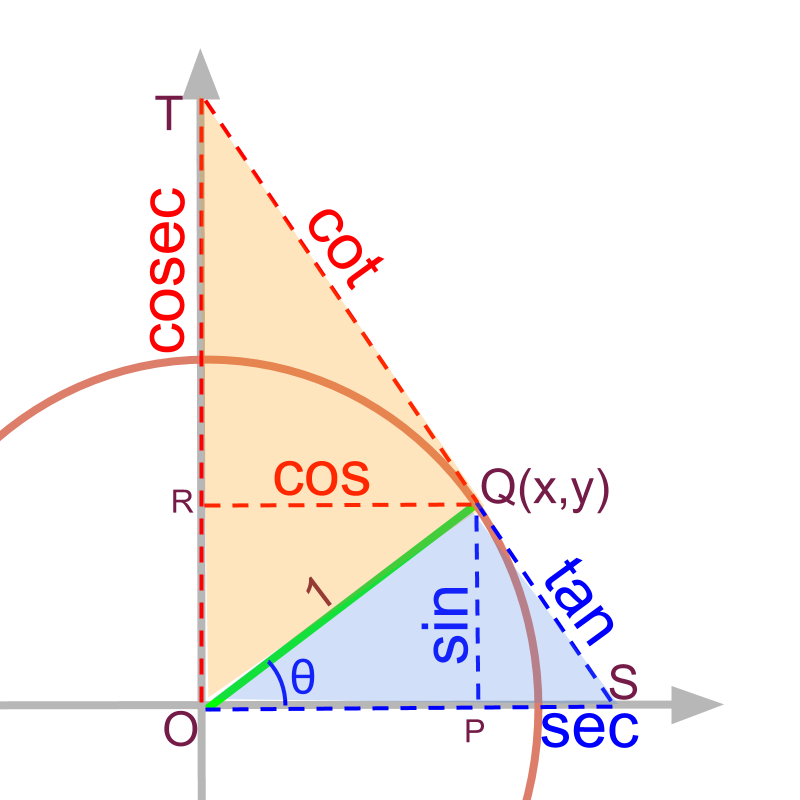The trigonometric value corresponding to $\overline{QT}$$\overline{Q T}$ is $\mathrm{cot}\theta$$\cot \theta$.

$\text{cot}\theta =\frac{1}{\mathrm{tan}\theta }=\frac{\mathrm{cos}\theta }{\mathrm{sin}\theta }=\frac{x}{y}$$\textrm{\cot} \theta = \frac{1}{\tan \theta} = \frac{\cos \theta}{\sin \theta} = \frac{x}{y}$

trigonometric values

The trigonometric values for a given angle $\theta$$\theta$ is defined as lengths in relation to

•  chord or sine : $\mathrm{sin}\theta$$\sin \theta$

•  tangent : $\mathrm{tan}\theta$$\tan \theta$

•  secant : $\mathrm{sec}\theta$$\sec \theta$

all the above for complementary angle

•  co-sine : $\mathrm{cos}\theta$$\cos \theta$

•  co-tangent : $\mathrm{cot}\theta$$\cot \theta$

•  co-secant : $\mathrm{csc}\theta$$\csc \theta$ or $\text{cosec}\theta$$\textrm{\cos e c} \theta$

summaryTrigonometric Values: For a line segment of unit length at the angle $\theta$$\theta$

The point on unit circle for given angle $\theta$$\theta$ is $P\left(x,y\right)$$P \left(x , y\right)$.

Note: $x$$x$ and $y$$y$ are the projections on $x$$x$-axis and $y$$y$-axis.

•  chord or sine : $\mathrm{sin}\theta =y$$\sin \theta = y$

•  tangent : $\mathrm{tan}\theta =\frac{y}{x}$$\tan \theta = \frac{y}{x}$

•  secant : $\mathrm{sec}\theta =\frac{1}{x}$$\sec \theta = \frac{1}{x}$

For complementary-angle

•  co-sine : $\mathrm{cos}\theta =x$$\cos \theta = x$

•  co-tangent : $\mathrm{cot}\theta =\frac{x}{y}$$\cot \theta = \frac{x}{y}$

•  co-secant : $\mathrm{csc}\theta$$\csc \theta$ or $\text{cosec}\theta =\frac{1}{y}$$\textrm{\cos e c} \theta = \frac{1}{y}$

Outline

It is advised to do the firmfunda version of "basics of Trigonometry" course before doing this.

The outline of material to learn "Advanced Trigonometry" is as follows.
Note: go to detailed outline of Advanced Trigonometry

→   Unit Circle form of Trigonmetric Values

→   Trigonometric Values in all Quadrants

→   Trigonometric Values or any Angles : First Principles

→   Understanding Trigonometric Values in First Quadrant

→   Trigonometric Values in First Quadrant

→   Trigonometric Values of Compound Angles: Geometrical Proof

→   Trigonometric Values of Compound Angles: Algebraic Proof

→   Trigonometric Values of Compound Angles: tan cot

→   Trigonometric Values of Compound Angles: more results